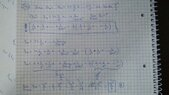# Limit of a sum

• MHB
Vali
I have the following sequence $(x_{n})$ , $x_{n}=1+\frac{1}{2^{2}}+...+\frac{1}{n^{2}}$ which has the limit $\frac{\pi ^{2}}{6}$.I need to calculate the limit of the sequence $(y_{n})$, $y_{n}=1+\frac{1}{3^{2}}+...+\frac{1}{(2n-1)^{2}}$
I don't know how to start.I think I need to solve the limit for all the sequence ( even n + odd n) then from the "big limit" I should subtract $\frac{\pi ^{2}}{6}$.How to start?

skeeter
I have the following sequence $(x_{n})$ , $x_{n}=1+\frac{1}{2^{2}}+...+\frac{1}{n^{2}}$ which has the limit $\frac{\pi ^{2}}{6}$.I need to calculate the limit of the sequence $(y_{n})$, $y_{n}=1+\frac{1}{3^{2}}+...+\frac{1}{(2n-1)^{2}}$
I don't know how to start.I think I need to solve the limit for all the sequence ( even n + odd n) then from the "big limit" I should subtract $\frac{\pi ^{2}}{6}$.How to start?

$x_n$ and $y_n$ are series ... sums of a sequence.

$\left(1 + \dfrac{1}{2^2} + \dfrac{1}{3^2} + ... + \dfrac{1}{n^2} + ... \right)-\left(1 + \dfrac{1}{3^2} + \dfrac{1}{5^2} + ... + \dfrac{1}{(2n-1)^2} + ... \right) = \dfrac{1}{2^2} + \dfrac{1}{4^2} + \dfrac{1}{6^2} + ... + \dfrac{1}{(2n)^2} + \, ...$

$\displaystyle \sum_{n=1}^\infty \dfrac{1}{n^2} - \sum_{n=1}^\infty \dfrac{1}{(2n-1)^2} = \sum_{n=1}^\infty \dfrac{1}{(2n)^2} = \dfrac{1}{4} \sum_{n=1}^\infty \dfrac{1}{n^2} = \dfrac{1}{4} \cdot \dfrac{\pi^2}{6} = \dfrac{\pi^2}{24}$

$\displaystyle \text{note} \implies \sum_{n=1}^\infty \dfrac{1}{n^2} - \sum_{n=1}^\infty \dfrac{1}{(2n)^2} = \sum_{n=1}^\infty \dfrac{1}{(2n-1)^2}$

Vali
Hi!
Thank you for the response!I found a solution, I got $\frac{\pi ^2}{8}$
View attachment 8794

#### Attachments

•tumblr_messaging_pmks4ed8My1tyt8xs_1280.jpg
82.5 KB · Views: 23